广度优先遍历（思路）

841. 钥匙和房间

解法一：广度优先(BFS)

class Solution {
//标志位就是防止被重复遍历；
//设置0号访问过，为了防止节点多次入队，需要在入队前将其设置为已访问；
//0号房间入队；
//没有被访问过；
//保证入队的都是没有被遍历过的；

}

体会

• 1.只要是广度优先的，肯定要用队列，天然是在一起的。 一边弹出，一边遍历，弹出一个以后，就把它的孩子或者是它的关联入队，保证把这一层，或这个节点的都弹出了，接着继续弹下一层或者下一个节点的。

• 2.树的BFS：先把root节点入队，然后再一层一层的遍历。
图的BFS也是一样的，与树的BFS的区别是：

• 1.树只有一个root，而图可以有多个源点，所有首先需要将多个源点入队。
• 2.树是有向的因此不需要标志是否访问过，而对于无向图而言，必须得标志是否访问过！并且为了防止某个节点多次入队，需要在入队前将其设置为已访问！
• 3.树用DFS比较多，图用BFS比较多；

133. 克隆图

题目描述

class Node {
public int val;
public List<Node> neighbors;
}

示例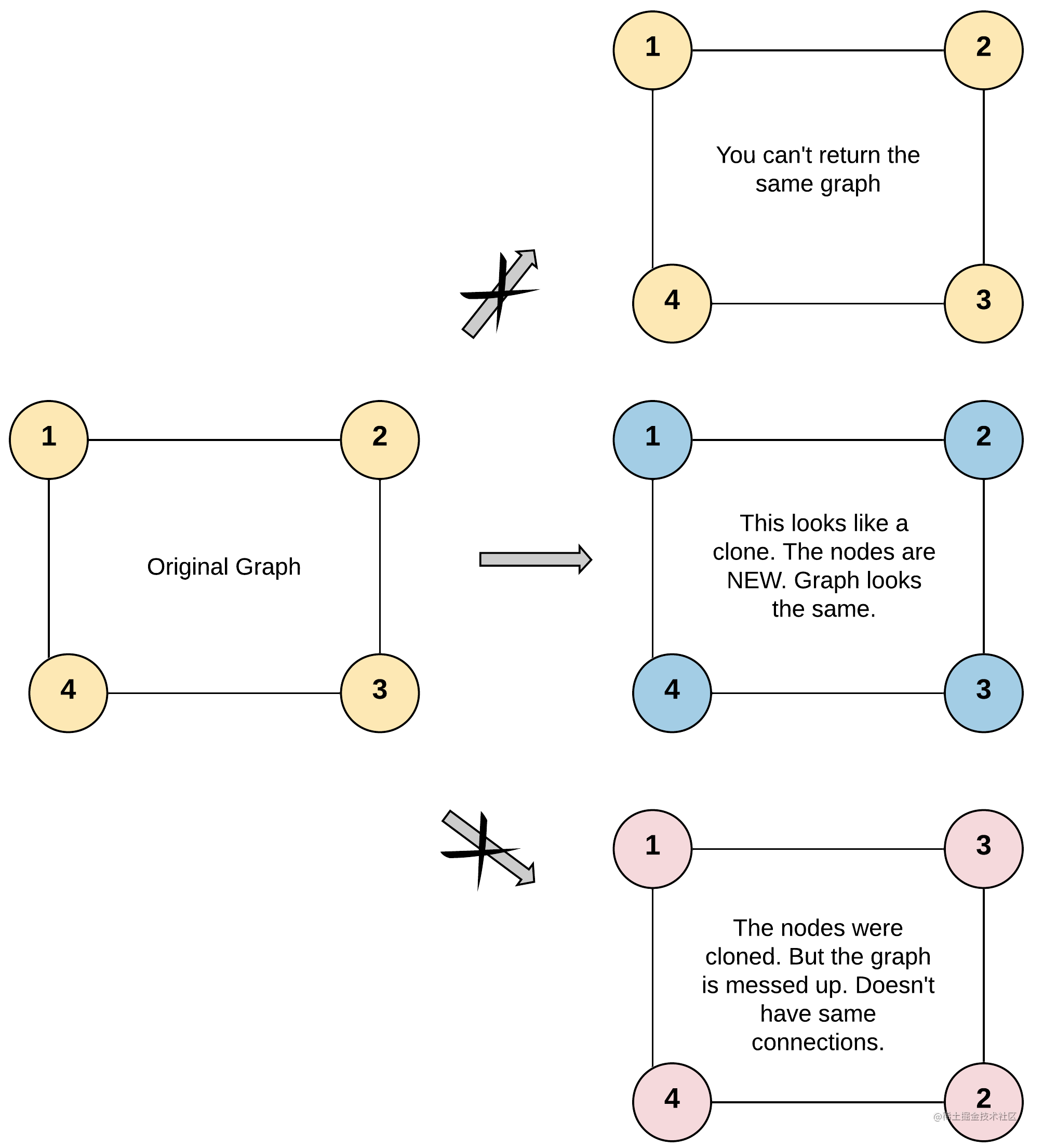解法一：广度优先(BFS)

// Definition for a Node.
class Node {
public int val;
public List<Node> neighbors;
public Node() {
val = 0;
neighbors = new ArrayList<Node>();
}
public Node(int _val) {
val = _val;
neighbors = new ArrayList<Node>();
}
public Node(int _val, ArrayList<Node> _neighbors) {
val = _val;
neighbors = _neighbors;
}
}

class Solution {
//首节点入队；
//克隆节点并入表；
//依次设置访问过并入队；
//添加邻居，注意是添加的克隆的；

}

1162. 地图分析

题目描述

示例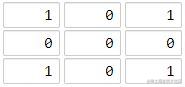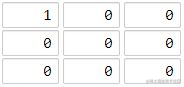解法一：广度优先(BFS)

1.树只有一个root，而图可以有多个源点，所有首先需要将多个源点入队。
2.树是有向的因此不需要标志是否访问过，而对于无向图而言，必须得标志是否访问过！并且为了防止某个节点多次入队，需要在入队前将其设置为已访问

class Solution {

//把所有的陆地先入队；
//设置四个变化方向；

//没有陆地或海洋；
//从各个陆地一圈一圈向外扩，最后就是最远的；
//出队点；

//求出此坐标的四个相邻坐标；
//剔除无效坐标和周围陆地；
//向外扩；
//返回最后一次出队的坐标点；

}

• 格子 (r, c) 的相邻四个格子为：(r-1, c)(r+1, c)(r, c-1) 和 (r, c+1)
• 使用函数 inArea 判断当前格子的坐标是否在网格范围内；
• 将遍历过的格子标记为 2，避免重复遍历。

// 网格结构的层序遍历
// 从格子 (i, j) 开始遍历
void bfs(int[][] grid, int i, int j) {
Queue<int[]> queue = new ArrayDeque<>();
while (!queue.isEmpty()) {
int n = queue.size();
for (int i = 0; i < n; i++) {
int[] node = queue.poll();
int r = node;
int c = node;
if (r-1 >= 0 && grid[r-1][c] == 0) {
grid[r-1][c] = 2;
}
if (r+1 < N && grid[r+1][c] == 0) {
grid[r+1][c] = 2;
}
if (c-1 >= 0 && grid[r][c-1] == 0) {
grid[r][c-1] = 2;
}
if (c+1 < N && grid[r][c+1] == 0) {
grid[r][c+1] = 2;
}
}
}
}

• 队列中的元素类型是 int[] 数组，每个数组的长度为 2，包含格子的行坐标和列坐标。
• 为了避免重复遍历，这里使用到了和 DFS 遍历一样的技巧：把已遍历的格子标记为 2。注意：我们在将格子放入队列之前就将其标记为 2。想一想，这是为什么？
• 在将格子放入队列之前就检查其坐标是否在网格范围内，避免将「不存在」的格子放入队列。

int[][] moves = {
{-1, 0}, {1, 0}, {0, -1}, {0, 1},
};

for (int[][] move : moves) {
int r2 = r + move;
int c2 = c + move;
if (inArea(grid, r2, c2) && grid[r2][c2] == 0) {
grid[r2][c2] = 2;
}
}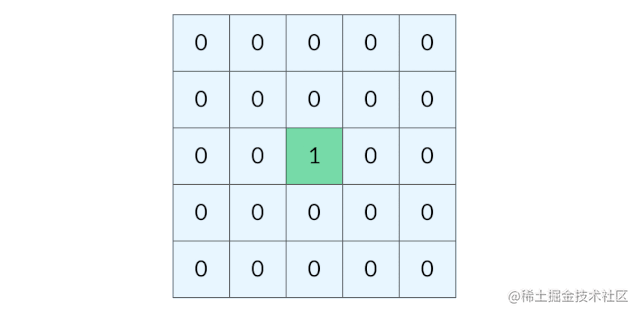BFS 完全可以以多个格子同时作为起点。我们可以把所有的陆地格子同时放入初始队列，然后开始层序遍历，这样遍历的效果如下图所示：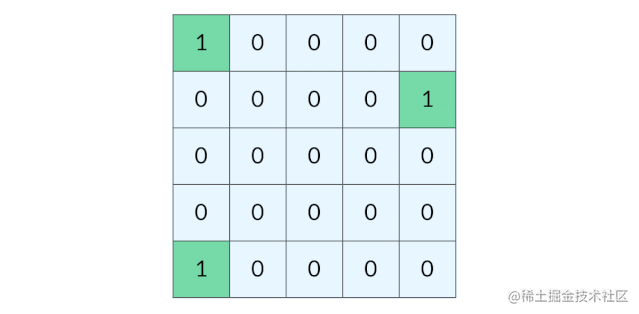*// 将所有的陆地格子加入队列*

*// 如果地图上只有陆地或者海洋，返回 -1*

*// 记录当前遍历的层数（距离）*

*// 判断坐标 (r, c) 是否在网格中*

体会

1.树只有一个root，而图可以有多个源点，所有首先需要将多个源点入队。
2.树是有向的因此不需要标志是否访问过，而对于无向图而言，必须得标志是否访问过！并且为了防止某个节点多次入队，需要在入队前将其设置为已访问！

752. 打开转盘锁

解法一：BFS

BFS算法框架：

//计算起点start到终点target的最小距离
int BFS(Node start, Node target){
//核心结构：队列；
//在图中都会用到，因为存在着交叉，会走回头路，树中则不需要，因为有next指针；
//起点入队；

//记录扩散步数；

//从当前队列中所有节点向与其关联的节点扩散；

//注意不同题目里这里的判断条件，是否到达终点；

//这里指的是当前节点的邻居节点；
//还没走过再加入；不走回头路；

//注意在这里更新步数；

}

class Solution {

//记录所有已经遍历到的密码；

//截止条件；

//处理相邻节点；一共8个；

}